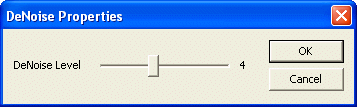# DeNoise Filter

The DeNoise filter reduces noise in the frames by averaging a number of frames.

## Using the DeNoise Filter

The DeNoise filter is loaded by an application using the following code:

## Parameters

The only parameter of the DeNoise filter is the DeNoise Level:

DeNoise Level
The parameter DeNoise Level determines the number of frames to be averaged.
Note: The actual number of frames that are averaged is 2^n, so when setting DeNoise Level to 2, 4 frames are averaged.
This parameter can only be set while the live video stream is stopped.

## Property Dialog

The number of frames to be averaged can be adjusted in the filter's property dialog:## Programmatically Access the Parameter

The following source code can be used, if the parameter should be set by an application:

<< IC Imaging Control Standard Filters